# Usage¶

To use phuzzy in a project:

import phuzzy
tn = phuzzy.TruncNorm(alpha0=[2, 3], alpha1=[], number_of_alpha_levels=15, name="t")
tri = phuzzy.Triangle(alpha0=[1, 4], alpha1=, number_of_alpha_levels=5)
f = tn + tri
print(f.df)


## available shapes¶

### Uniform¶

 1 2 3 import phuzzy.mpl as phm uni = phm.Uniform(alpha0=[1, 4], number_of_alpha_levels=5, name="x") uni.plot(show=True, filepath="/tmp/uniform.png", title=True)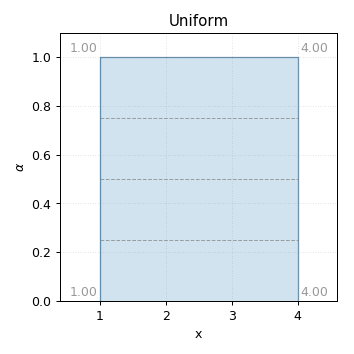Uniform fuzzy number (this is just an interval)

### Triangle¶

 1 2 3 4 import phuzzy.mpl as phm tri = phm.Triangle(alpha0=[1, 4], alpha1=, number_of_alpha_levels=5) tri.plot(show=False, filepath="/tmp/triangle.png", title=True) 

### Trapezoid¶

 1 2 3 import phuzzy.mpl as phm trap = phm.Trapezoid(alpha0=[1, 5], alpha1=[2, 3], number_of_alpha_levels=5) trap.plot(show=False, filepath="/tmp/trapezoid.png", title=True) 

### TruncNorm¶

 1 2 3 import phuzzy.mpl as phm tn = phm.TruncNorm(alpha0=[1, 3], number_of_alpha_levels=15, name="x") tn.plot(show=False, filepath="/tmp/truncnorm.png", title=True) 

### TruncGenNorm¶

 1 2 3 import phuzzy.mpl as phm tgn = phm.TruncGenNorm(alpha0=[1, 4], alpha1=[2, 3], number_of_alpha_levels=15, beta=3.) tgn.plot(show=False, filepath="/tmp/truncgennorm.png", title=True) 

### Superellipse¶

 1 2 3 import phuzzy.mpl as phm se = phm.Superellipse(alpha0=[-1, 2.], alpha1=None, m=1.0, n=.5, number_of_alpha_levels=17) se.plot(show=True, filepath="/tmp/superellipse.png", title=True) 

## basic operations¶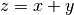1 2 3 4 x = phuzzy.Trapezoid(alpha0=[0, 4], alpha1=[2, 3], number_of_alpha_levels=5) y = phuzzy.TruncNorm(alpha0=[1, 3], number_of_alpha_levels=15, name="y") z = x + y z.name = "x+y"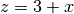1 2 3 x = phuzzy.Trapezoid(alpha0=[0, 4], alpha1=[2, 3], number_of_alpha_levels=5) z = 3 + x z = x + 3 

### Substraction¶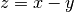1 2 3 4 x = phuzzy.Trapezoid(alpha0=[0, 4], alpha1=[2, 3], number_of_alpha_levels=5) y = phuzzy.TruncNorm(alpha0=[1, 3], number_of_alpha_levels=15, name="y") z = x - y z.name = "x-y"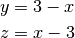1 2 3 x = phuzzy.Trapezoid(alpha0=[0, 4], alpha1=[2, 3], number_of_alpha_levels=5) y = 3 - x z = x - 3 

### Multiplication¶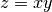1 2 3 4 x = phuzzy.Trapezoid(alpha0=[0, 4], alpha1=[2, 3], number_of_alpha_levels=5) y = phuzzy.TruncNorm(alpha0=[1, 3], number_of_alpha_levels=15, name="y") z = x * y z.name = "x*y"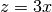1 2 3 x = phuzzy.Trapezoid(alpha0=[0, 4], alpha1=[2, 3], number_of_alpha_levels=5) z = 3 * x z = x * 3 

### Division¶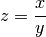1 2 3 4 x = phuzzy.Trapezoid(alpha0=[0, 4], alpha1=[2, 3], number_of_alpha_levels=5) y = phuzzy.TruncNorm(alpha0=[1, 3], number_of_alpha_levels=15, name="y") z = x / y z.name = "x/y"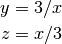1 2 3 x = phuzzy.Trapezoid(alpha0=[0, 4], alpha1=[2, 3], number_of_alpha_levels=5) z = 3 / x z = x / 3 

### Exponentiation¶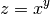1 2 3 4 x = phuzzy.Trapezoid(alpha0=[0, 4], alpha1=[2, 3], number_of_alpha_levels=5) y = phuzzy.TruncNorm(alpha0=[1, 3], number_of_alpha_levels=15, name="y") z = x ** y z.name = "x^y"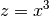1 2 x = phuzzy.Trapezoid(alpha0=[0, 4], alpha1=[2, 3], number_of_alpha_levels=5) z = x**3 

### Negation¶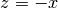1 2 x = phuzzy.Trapezoid(alpha0=[0, 4], alpha1=[2, 3], number_of_alpha_levels=5) z = -x 

### Absolute value¶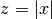1 2 3 x = phuzzy.Trapezoid(alpha0=[-1, 4], alpha1=[2, 3], number_of_alpha_levels=5) z = abs(x) z = x.abs()